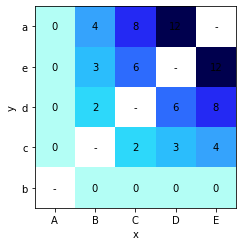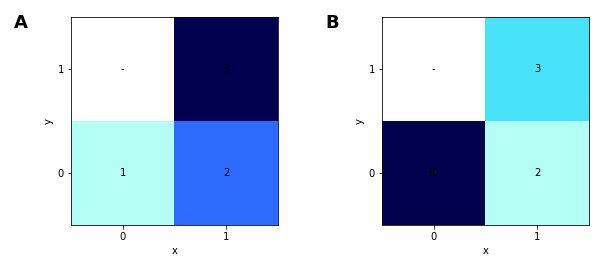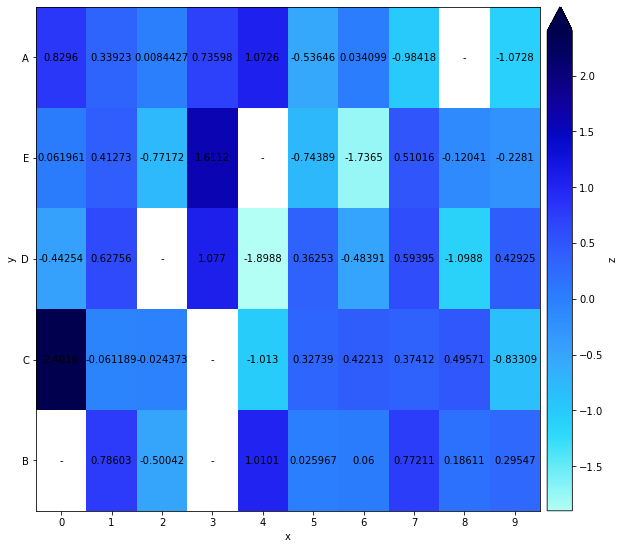# HeatMap#

Title
HeatMap Element
Dependencies
Matplotlib
Backends
Matplotlib
Bokeh
Plotly
```import numpy as np
import holoviews as hv

hv.extension('matplotlib')
````HeatMap` visualises tabular data indexed by two key dimensions as a grid of colored values. This allows spotting correlations in multivariate data and provides a high-level overview of how the two variables are plotted.

The data for a `HeatMap` may be supplied as 2D tabular data with one or more associated value dimensions. The first value dimension will be colormapped, but further value dimensions may be revealed using the hover tool.

```data = [(chr(65+i), chr(97+j),  i*j) for i in range(5) for j in range(5) if i!=j]
hv.HeatMap(data).sort()
```It is important to note that the data should be aggregated before plotting as the `HeatMap` cannot display multiple values for one coordinate and will simply use the first value it finds for each combination of x- and y-coordinates.

```heatmap = hv.HeatMap([(0, 0, 1), (0, 0, 10), (1, 0, 2), (1, 1, 3)])
heatmap + heatmap.aggregate(function=np.max)
```
```WARNING:param.HeatMapPlot01524: HeatMap element index is not unique,  ensure you aggregate the data before displaying it, e.g. using heatmap.aggregate(function=np.mean). Duplicate index values have been dropped.
```As the above example shows before aggregating the second value for the (0, 0) is ignored unless we aggregate the data first.

To reveal the values of a `HeatMap` we can enable a `colorbar` and if you wish to have interactive hover information, you can use the hover tool in the Bokeh backend.

Note that a HeatMap allows mixtures of categorical, numeric and datetime values along the x- and y-axes:

```heatmap = hv.HeatMap((np.random.randint(0, 10, 100), np.random.choice(['A', 'B', 'C', 'D', 'E'], 100),
np.random.randn(100), np.random.randn(100)), vdims=['z', 'z2']).sort().aggregate(function=np.mean)

heatmap.opts(colorbar=True, fig_size=250, clim=(-2, 2))
```For full documentation and the available style and plot options, use `hv.help(hv.HeatMap).`

This web page was generated from a Jupyter notebook and not all interactivity will work on this website. Right click to download and run locally for full Python-backed interactivity.